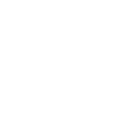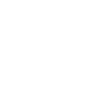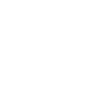正文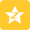Qzone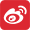微博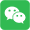微信

陕西食药监局：13批次食品抽检不合格 涉天佑食品

2017-12-06 18:08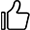1.豆制品1批次，大同大润发商业有限公司销售的标称河北孙老大食品有限公司生产的火腿味香干(素食)铅超标，检出值为0.9mg/kg，标准规定为≤0.5mg/kg。

2.乳制品1批次，阳曲县瑞美乳业有限公司生产的淳真牛奶菌落总数超标，检出值为n1＜10,n2=1.1×106, n3=1.1×106,n4=7.5×105,n5=1.2×106(CFU/mL)，标准规定为n=5,c=2,m=50000,M=100000(CFU/mL)。

3.酒类1批次，洪洞县华尧纯酿私藏酒业销售的标称安徽晨野酒业有限公司生产的古玉贵宾酒(浓香型白酒)酒精度不符合标签明示值要求，酒精度检出值为42.1%voL,标签明示值为46±1.0%voL。

4.饮料10批次。太原市崛围泉饮品有限公司生产的饮用纯净水检出铜绿假单胞菌,检出值为n1=0,n2=0,n3=16,n4=89,n5=21(CFU/250mL),标准规定为n=5,c=0,m=0(CFU/250mL)。

5.平遥县盛慈饮品有限公司生产的盛慈饮用纯净水检出铜绿假单胞菌,检出值为n1=58,n2=65, n3=46,n4=86,n5=68(CFU/250mL)，标准规定为n=5,c=0,m=0(CFU/250mL)。

6.平遥县天佑食品有限公司生产的包装饮用水检出铜绿假单胞菌,检出值为n1=0,n2=0,n3=0, n4=2,n5=0(CFU/250mL)，标准规定为n=5,c=0,m=0(CFU/250mL)。

7.河津市城区办金玉五度桶装水经销部销售的标称山西德润福食品股份有限公司生产的饮用纯净水检出铜绿假单胞菌,检出值为n1=79,n2=0,n3=0 ，n4=0,n5=0(CFU/250mL)，标准规定为n=5,c=0,m=0(CFU/250mL)。

8.河津市晋美源饮用水厂生产的饮用纯净水检出铜绿假单胞菌,检出值为n1=2,n2=0,n3=0, n4=0,n5=0(CFU/250mL)，标准规定为n=5,c=0,m=0(CFU/250mL)。

9.垣曲县源源饮用水厂生产的饮用纯净水检出铜绿假单胞菌,检出值为n1=6,n2=21,n3=26,n4=86,n5=5(CFU/250mL)标准规定为n=5,c=0,m=0(CFU/250mL)。

10.万荣县鑫水源饮用水有限公司生产的桶装饮用纯净水检出铜绿假单胞菌,检出值为n1=10,n2=10,n3=4,n4=9,n5=4(CFU/250mL)，标准规定为n=5,c=0,m=0(CFU/250mL)。

11.晋中天怡山泉纯净水有限公司生产的桶装纯净饮用水检出铜绿假单胞菌,检出值为n1=5,n2=97,n3=65,n4=8,n5=0(CFU/250mL)，标准规定为n=5,c=0,m=0(CFU/250mL)。

12.太原市东龙天泉水业有限公司生产的包装饮用水(其他饮用水)检出铜绿假单胞菌,检出值为n1=0,n2=69, n3=84,n4=0,n5=4(CFU/250mL)，标准规定为n=5,c=0,m=0(CFU/250mL)。

13.山西冰泉饮品有限公司生产的桶装饮用水检出铜绿假单胞菌,检出值为n1=92,n2=76,n3=39,n4=79,n5=61(CFU/250mL)，标准规定为n=5,c=0,m=0(CFU/250mL)。